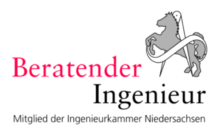## The nominal stress developed at global level

The nominal stress concept (NSC) is the most commonly used method for assessing fatigue strength for metallic structures. The nominal stress of the considered component is assumed as the characteristic value of existing and bearable stresses. If this component shows significant cross-sectional changes, there are areas with stress concentrations due to the geometrical changes. Nevertheless, in case of a static design the nominal stresses are still assumed based on the net cross-sectional area because in the ultimate limit state the stress peaks are usually reduced by a plastic rearrangement. However, this is not the case for dynamic loads. Therefore, local stress peaks due to structural discontinuities have to be considered.

On the nominal stress concept (global approach) are detected these surges in contrast to the local approach not constructed, but on the resistance side of the assessed structural details. Therefore be needed for the application of the rated stress concept component Wöhler lines based on the basis of experiments. These are named in honor of WOEHLER because he carried out the first systematic experimental studies under cyclic loading in the years around 1860.

In the WÖHLER test, the following are measured on different voltage horizons with a single-stage nominal voltage oscillation amplitude ∆σN and specified stress ratio (∆σm = const. or R = const.) several vibration tests were carried out and the number of vibration cycles N up to the defined failure was determined. Since the test results are subject to very large scattering, it is assumed in the evaluation that the logarithms of the test results (∆σN and N) can be determined by a Gaussian normal distribution.

Usually a survival probability PÜ of 95% is specified, which is bound to a certain confidence probability regarding the evaluation of the test results.

In general, the Woehler diagram is divided into the following three areas:

• Short term strength (Low Cycle Fatigue, LCF):
• Fatigue strength:
• Fatigue strength (High Cycle Fatigue, HCF):

Range for N < 104

Range between N =104 to 107

Range for N > 107

The SKI engineering company has opportunities to test welded connections and wolted connections bis in den Giga-Cycle-Fatigue Bereich (GCF) zu testen, d.h. bis zu 109 Schwingspielen mit konstanter oder variabler Amplitude.

In the WÖHLER test, a single-stage nominal voltage oscillation width ∆σN and specified stress ratio (∆σm = const. or R = const.) several vibration tests were performed and the number of vibration cycles N up to the defined failure was determined. Since the test results are subject to very large scatter, it is assumed for the evaluation that the logarithms of the test results (∆σN and N) can be captured by a Gaussian normal distribution.SKI Ingenieurges. mbH
Mengendamm 12
30177 Hannover

Phone: +49 (0) 511 / 261847-0

## Consulting Engineers